Like   Tweet   Pin   +1   in
 /* styles */ Tai Chi Stories, Tips and Resources   ||   Issue #024 March 2020
 table div table+table+table div table{width:100%;padding:0}table div table+table+table div table img{width:96.23%;padding:0;float:none}table div table+table+table div table td{width:100%;padding:0 1.88% 18px}/* styles */Welcome to the March issue of Good Chi News. I hope you are all safe and well.

In this issue I've included a YouTube clip on the benefits of Tai Chi by a taoist monk.

Also included is our regular Tai Chi tip, a Lao Tzu quote and a couple of reflections - one on the corona virus and the other on the value of research and practice.

Regards
Chris Bennett
Chris Chi

 table div table+table+table+table+table div table{width:100%;padding:0}table div table+table+table+table+table div table img{width:96.23%;padding:0;float:none}table div table+table+table+table+table div table td{width:100%;padding:0 1.88% 18px}/* styles */## Crisis and Opportunity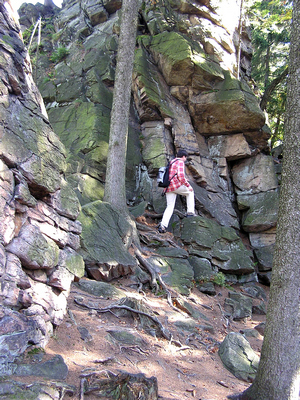Well, the corona virus pandemic has certainly bought a halt to all my face to face training workshops.

I've had several cancellations to my local regular Chair Chi sessions in various aged care centres. And rightly so as it makes sense to reduce the risk of the spread of the infection by restricting access to aged care centres for non essential services.

So until the crisis eases I'm directing my efforts to creating online courses and will also spend the extra time available to complete the third draft of my memoir. And also tidy up the garden :)

 table div table+table+table+table+table+table+table+table div table{width:100%;padding:0}table div table+table+table+table+table+table+table+table div table img{width:96.23%;padding:0;float:none}table div table+table+table+table+table+table+table+table div table td{width:100%;padding:0 1.88% 18px}/* styles */## Tai Chi Tip

 /* styles */ After you have learned a Tai Chi form try and focus on the Yi (the mind) leading the Chi (energy/movement)
 table div table+table+table+table+table+table+table+table+table+table+table div table{width:100%;padding:0}table div table+table+table+table+table+table+table+table+table+table+table div table img{width:96.23%;padding:0;float:none}table div table+table+table+table+table+table+table+table+table+table+table div table td{width:100%;padding:0 1.88% 18px}/* styles */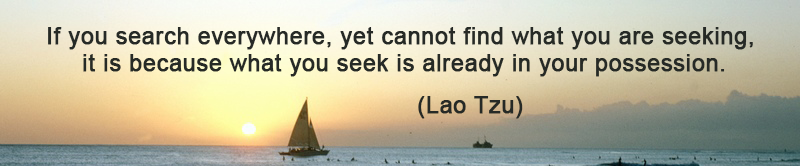table div table+table+table+table+table+table+table+table+table+table+table+table+table div table{width:100%;padding:0}table div table+table+table+table+table+table+table+table+table+table+table+table+table div table img{width:96.23%;padding:0;float:none}table div table+table+table+table+table+table+table+table+table+table+table+table+table div table td{width:100%;padding:0 1.88% 18px}/* styles */## Research + Practice = Improvement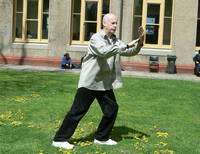One of the personal benefits of developing a new program like Sports Chi is that it gives me the opportunity to research and cross reference various Tai Chi themes and techniques.

Not only is this research beneficial for my Sports Chi program but also for my own personal training. Read More ...

 table div table+table+table+table+table+table+table+table+table+table+table+table+table+table+table+table div table{width:100%;padding:0}table div table+table+table+table+table+table+table+table+table+table+table+table+table+table+table+table div table img{width:96.23%;padding:0;float:none}table div table+table+table+table+table+table+table+table+table+table+table+table+table+table+table+table div table td{width:100%;padding:0 1.88% 18px}/* styles */## The Benefits of Tai Chi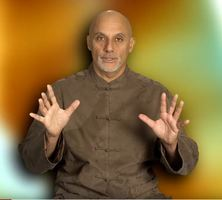In this 11.26 YouTube Clip Taoist monk Yunrou discusses the theory of Chi and it's effect on the body and mind.

He says that 'Tai Chi is interesting and intricate thing and ... it's not boring' which helps motivate people to exercise. He also covers the topic of stress which is very interesting.

 table div table+table+table+table+table+table+table+table+table+table+table+table+table+table+table+table+table+table+table div table{width:100%;padding:0}table div table+table+table+table+table+table+table+table+table+table+table+table+table+table+table+table+table+table+table div table img{width:96.23%;padding:0;float:none}table div table+table+table+table+table+table+table+table+table+table+table+table+table+table+table+table+table+table+table div table td{width:100%;padding:0 1.88% 18px}/* styles */## Training: One Leg Stance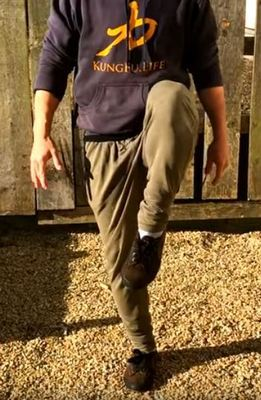Occasionally I like to share skills from other arts such as Kung Fu that can be beneficial to your health and wellbeing. I choose skills that include Tai Chi principles such as relaxation, correct structure, balance, etc.

Here's a handy sequence of single leg exercises to strengthen your legs and other parts of the body from Master Yan Xin. I've incorporated a similar routine into my personal training.

The exercises are clearly explained and easy to follow. Make sure you do what you can and build from there.

 table div table+table+table+table+table+table+table+table+table+table+table+table+table+table+table+table+table+table+table+table+table+table div table{width:100%;padding:0}table div table+table+table+table+table+table+table+table+table+table+table+table+table+table+table+table+table+table+table+table+table+table div table img{width:96.23%;padding:0;float:none}table div table+table+table+table+table+table+table+table+table+table+table+table+table+table+table+table+table+table+table+table+table+table div table td{width:100%;padding:0 1.88% 18px}/* styles *//* styles */
 table div table+table+table+table+table+table+table+table+table+table+table+table+table+table+table+table+table+table+table+table+table+table+table+table div table{width:100%;padding:0}table div table+table+table+table+table+table+table+table+table+table+table+table+table+table+table+table+table+table+table+table+table+table+table+table div table img{width:96.23%;padding:0;float:none}table div table+table+table+table+table+table+table+table+table+table+table+table+table+table+table+table+table+table+table+table+table+table+table+table div table td{width:100%;padding:0 1.88% 18px}/* styles */